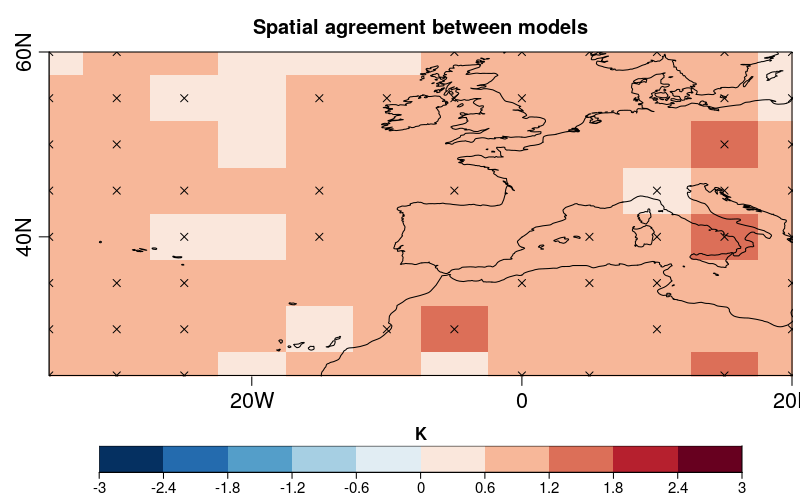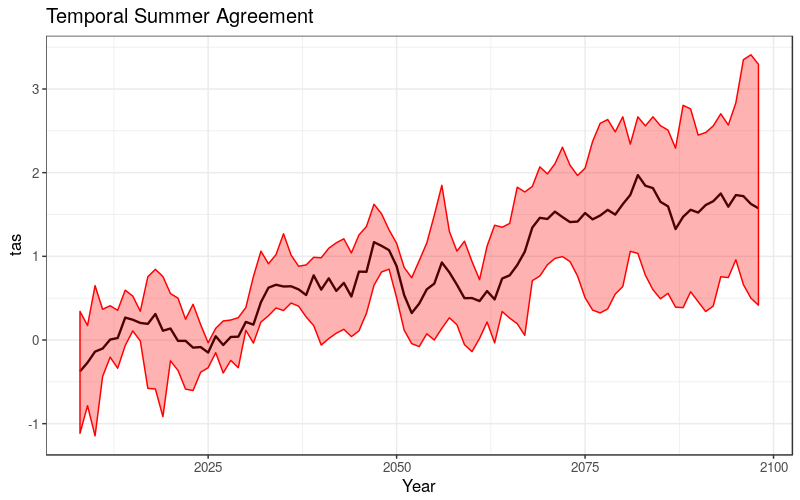# Multi-model agreement

Multi-model agreement performs a comparison of climate model projections anomalies. This vignette illustrates step-by-step how to perform a multi-model agreement assessment using ClimProjDiags package functionalities. The following example simulates the case of summer projections temperature anomalies for different models.

This example requires the following system libraries:

• libssl-dev
• libnecdf-dev
• cdo

The ClimProjDiags R package should be loaded by running the following lines in R, onces it is integrated into CRAN mirror.

``````library(ClimProjDiags)
``````

All the other R packages involved can be installed directly from CRAN and loaded as follows:

``````library(abind)
library(s2dverification)
library(ggplot2)
``````

### 2- Define the problem and the correspondent data and parameters

The aim is to know, compared with a reference period:

• what is the sign of the future anomaly for a certain climate variable, and
• what is the percentage of models projecting this anomaly

The ilustrative problem is to compare the monthly mean air temperature at 2 m in summer between four different models. The reference period used from the historical simulations to perform the anomalies is 1961 - 1990. While, the future scenario chosen is the rcp2.6 during the period 2006 - 2100. Finally, the region selected in the northern hemisphere is between -40 - 20 ºE and 25 - 60 ºN.

The parameters are defined by running the next lines in R:

``````var <- 'tas'

start_climatology <- '1961'
end_climatology <- '1990'

start_projection <- '2006'
end_projection <- '2100'

lat <- seq(25, 60, 5)
lon <- seq(-35, 20 ,5)
``````

A synthetic sample of data for the reference period is built by adding random perturbation to a sinusoidal function. The latitudinal behavior of the temperature is considered by subtracting randomly a value proportional to the latitude. Furthermore, attributes of time and dimensions are added.

``````multimodel_historical <- NULL
for (k in 1 : 4) {
grid1 <- 293 - 10 * cos(2 * pi / 12 * (1 : 360)) + rnorm(360)
gridlon <- NULL
for (i in 1 : 12) {
gridlon <- cbind(gridlon,
grid1 + rnorm(360, sd = 5) * cos(2 * pi / 12 * (1 : 360)))
}
gridpoint <- NULL
for (j in 1 : 8) {
gridnew <- apply(gridlon, 2, function(x) {x - rnorm(360, mean = j * 0.5,
sd = 3)})
gridpoint <- abind(gridpoint, gridnew, along = 3)
}
multimodel_historical <- abind(multimodel_historical, gridpoint, along = 4)
}
multimodel_historical <- InsertDim(multimodel_historical, posdim = 5, lendim = 1)
multimodel_historical <- aperm(multimodel_historical, c(4, 5, 1, 2, 3))
names(dim(multimodel_historical)) <- c("model", "var", "time", "lon", "lat")

time <- seq(ISOdate(1961, 1, 15), ISOdate(1990, 12, 15), "month")
metadata <- list(time = list(standard_name = 'time', long_name = 'time',
calendar = 'proleptic_gregorian',
units = 'days since 1970-01-01 00:00:00', prec = 'double',
dim = list(list(name = 'time', unlim = FALSE))))
attr(multimodel_historical, 'Variables')\$dat1\$time <- time
``````

A similar procedure is considered to build the synthetic data for the future projections. However, a small trend is added in order to make the data partially more realistic.

``````multimodel_projection <- NULL
for (k in 1 : 4) {
grid1 <- 293 - 10 * cos(2 * pi / 12 * (1 : 1140)) + rnorm(1140) +
(1 : 1140) * rnorm(1, mean = 1.5) / 1140
gridlon <- NULL
for (i in 1 : 12) {
gridlon <- cbind(gridlon,
grid1 + rnorm(1140, sd = 5) * cos(2 * pi / 12 * (1 : 1140)))
}
gridpoint <- NULL
for (j in 1 : 8) {
gridnew <- apply(gridlon, 2, function(x) {x - rnorm(1140, mean = j * 0.5,
sd = 3)})
gridpoint <- abind(gridpoint, gridnew, along = 3)
}
multimodel_projection <- abind(multimodel_projection, gridpoint, along = 4)
}
multimodel_projection <- InsertDim(multimodel_projection, posdim = 5, lendim = 1)
multimodel_projection <- aperm(multimodel_projection, c(4, 5, 1, 2, 3))
names(dim(multimodel_projection)) <- c("model", "var", "time", "lon", "lat")

time <- seq(ISOdate(2006, 1, 15), ISOdate(2100, 12, 15), "month")
metadata <- list(time = list(standard_name = 'time', long_name = 'time',
calendar = 'proleptic_gregorian',
units = 'days since 1970-01-01 00:00:00', prec = 'double',
dim = list(list(name = 'time', unlim = FALSE))))
attr(multimodel_projection, 'Variables')\$dat1\$time <- time
``````

Now, two objects called `multimodel_historical` and `multimodel_projection` are available in the R environment. A check can be done to the loaded data by comparing with the next lines (due to the random functions the results may differ between each execution):

``````> dim(multimodel_historical)
model   var  time   lon   lat
4     1   360    12    8

> summary(multimodel_historical)
Min. 1st Qu.  Median    Mean 3rd Qu.    Max.
251.2   281.7   287.9   287.8   294.0   321.6

> dim(multimodel_projection)
model   var  time   lon   lat
4     1  1140    12     8

> summary(multimodel_projection)
Min. 1st Qu.  Median    Mean 3rd Qu.    Max.
254.8   282.8   288.8   288.8   294.9   322.6
``````

### 3- Multi-model agreement based on seasonal analysis

The multi-model agreement is a comparison based on seasonal anomalies, which are computed by following the next steps:

• First, the desired season is selected for the reference data with the `SeasonSelect` function from ClimProjDiags package. In this case, the boreal summer is chosen by defining the parameter `season = 'JJA'`.
``````summer_historical <- SeasonSelect(multimodel_historical, season = 'JJA')
``````

The new subsets are lists of two elements. The first element is the selected data and the second element contains the corresponding dates.

``````> str(summer_historical)
List of 2
\$ data : num [1:4, 1:90, 1:12, 1:8] 314 293 305 300 300 ...
\$ dates: chr [1:90] "1961-06-15 12:00:00" "1961-07-15 12:00:00" ...

> dim(summer_historical\$data)
model  time   lon   lat
4    90    12     8
``````
• Now, the mean climatology value for each grid point and model can be computed by using the `Mean1Dim()` function belonging to the s2dverification package. The position of the temporal dimension should be specified in parameter `posdim`.
``````climatology <- Mean1Dim(summer_historical\$data, posdim = 2)
``````
• `Season()` function from s2dverification package returns the mean annual time series for the selected season by defining the parameters of the initial month of the data (`monini = 1`), the first month of the season (`moninf = 6`) and the final month of the season (`monsup = 8`). The last two parameters, `moninf` and `monsup`, have their origin with respect to the first one `monini`.
``````summer_projection <- Season(multimodel_projection, posdim = 3,
monini = 1, moninf = 6, monsup = 8)
``````

By running the next lines, it is possible to check the dimensions of the data:

``````> dim(climatology)
model   lon   lat
4    12     8
> dim(summer_projection)
model   var  time   lon   lat
4     1   95    12     8
``````
• A new dimension will be added by running the function `InsertDim()` in order to obtain the same dimensions as the projections data. `InsertDim()` repeats the original data the required number of times (21 years of future simulations) in the adequated position (the temporal dimension in the summer_projection data is in the third position).
``````climatology <- InsertDim(InsertDim(climatology, posdim = 2, lendim = 1),
posdim = 3, lendim = 95)
``````
• The anomaly for each model is obtained by simply subtracting.
``````anomaly <- summer_projection - climatology
``````

### 4- Multi-model agreement spatial visualitzation

In order to obtain a spatial visualitzation, the temporal mean is computed. So, the time average anomalies for all models is saved in the `average` object. `AnoAgree()` function from ClimProjDiags package calculates the percentages of models which agrees with a positive or negative mean in each grid point.

``````average <- Mean1Dim(anomaly,  which(names(dim(anomaly)) == "time"))
agreement <- AnoAgree(average, membersdim = which(names(dim(average)) == "model"))
``````

So, in a case when four models are being compared, the `agreement` object can take the following values: 100 (all models agree), 75 (only one model has opposite sign), 50 (only two models agree with the mean signal) and 25 (one model agrees with the sign of the mean signal because its magnitude is higher that the other three models). These values will change with the number of compared models.

The next question will be answered by the example plot: Where do 80 % or more models agree in the signal? To obtain this plot, the next lines should be run in R. Notice you can modify the threshold by modifying the parameter `agreement_threshold`. The colour map shows the mean temperature anomaly and the dots the model agreement. The plot will be saved with the name “SpatialSummerAgreement.png”.

``````agreement_threshold <- 80
colorbar_lim <- ceiling(max(abs(max(average)), abs(min(average))))
brks <- seq(-colorbar_lim, colorbar_lim, length.out = 11)

PlotEquiMap(drop(Mean1Dim(average, which(names(dim(average)) == "model"))),
lat = lat, lon = lon, units = "K", brks = brks,
toptitle = paste(var, "- climatology:", start_climatology, "to",
end_climatology, "and future simulation:",
start_projection, "to", end_projection),
filled.continents = FALSE, title_scale = 0.6,
dots = drop(agreement) >= agreement_threshold,
fileout = "SpatialSummerAgreement.png")
``````### 5- Multi-model agreement temporal visualization

To visualize the time evolution of multi-model agreement, the spatial average is performed by a grid pixel size using the `WeightedMean` function from the ClimProjDiags package. Also, a smooth filter is applied with the `Smoothing()` function from the s2dverifiction package. In this example, a 5-year moving window filter is applied by defining the parameter `runmeanlen = 5`.

``````temporal <- drop(WeightedMean(anomaly, lon = lon, lat = lat, mask = NULL))
temporal <- Smoothing(temporal, runmeanlen = 5, numdimt = 2)
``````

Before visualizing, a data frame with the proper format is created.

``````data_frame <- as.data.frame.table(t(temporal))
years <- rep(start_projection : end_projection, 4)
data_frame\$Year <- c(years)
names(data_frame) <- "Model"
for (i in 1 : length(levels(data_frame\$Model))) {
levels(data_frame\$Model)[i] <- paste0("model", i)
}
``````

A new png file will be saved in the working directory with the name “TemporalSummerAgreement.png”.

``````g <- ggplot(data_frame, aes(x = Year, y = Freq)) + theme_bw() +
ylab("tas") +  xlab("Year") + theme(text=element_text(size = 12),
legend.text=element_text(size = 12),
axis.title=element_text(size = 12)) +
stat_summary(data =  data_frame, fun.y= "mean",
mapping = aes(x = data_frame\$Year, y = data_frame\$Freq,
group = interaction(data_frame[2,3]),
color = data_frame\$Model),
geom = "line", size = 0.8) +
stat_summary(data =  data_frame, geom = "ribbon",
fun.ymin = "min", fun.ymax = "max",
mapping = aes(x = data_frame\$Year, y = data_frame\$Freq,
group = interaction(data_frame[2,3])),
alpha = 0.3, color = "red", fill = "red") +
ggtitle("Temporal Summer Agreement")
ggsave(filename = "TemporalSummerAgreement.png", g, device = NULL, width = 8,
height = 5, units = 'in', dpi = 100)
``````

Note: if a warning appears when plotting the temporal time series, it might be due to the NA's values introduced when smoothing the time series.